# 多智能体强化学习算法

## Neighborhood Cognition Consistent Multi-Agent Reinforcement Learning

Posted by MY on August 17, 2020

## 一、问题

• 对环境形成一致性的认知是实现良好协作的必要条件；
• 智能体只与具有局部感知的邻居智能体直接交互的事实表明，只要邻居智能体之间相互保持认知一致性通常足以保证系统级的协作。 受上述事实启发，本文首次提出了邻域认知一致性（Neighborhood Cognitive Consistency）的概念应用于多智能体研究。

## 二、解法

### 2.1 NCC-Q

#### 2.1.2 网络结构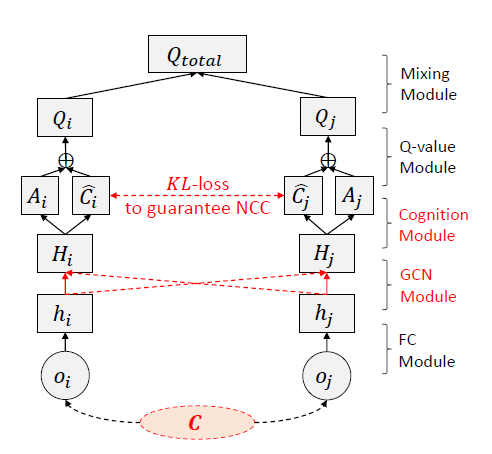• 全连接模块：将局部观察$o_i$编码为仅智能体特定认知的信息$h_i$
• GCN模块：将邻域中所有的$h_i$进行聚合,得到高维抽象$H_{i}=\sigma\left(W \Sigma_{j \in N(i) \cap{i}} \frac{h_{j}}{\sqrt{|N(j)||N(i)|}}\right)$。其中$N(i)$表示智能体$i$的邻居智能体。使用$\sqrt{|N(j)||N(i)|}$对$h_j$做标准化是为了降低出度（入度）高的邻居的影响。同一邻域内的所有智能体都采用同一个$W$以期望更容易达到邻域认知一致性。
• 认知模块：将$H_{i}$分解为两部分，分别是智能体特定认知表征$A_i$和邻域特定认知表征$\widehat{C_{i}}$。
• Q-value模块：将$A_i$与$\widehat{C_{i}}$求和作为输入得到Q值$Q_{i}\left(o_{i}, a_{i}\right)$
• 混合模块：使用VDN或QMIX的方式将所有$Q_{i}\left(o_{i}, a_{i}\right)$进行加权求和得到联合值函数$Q_{\text {total}}(\vec{o}, \vec{a})$

#### 2.1.3 邻域认知一致性

• 对于每一个邻域都有一个真实的认知隐变量$C$，并且从$C$可以得到邻域中每个智能体的局部观察。如下图所示，从邻域中的观察抽取出每个智能体的局部观察，相比于从全局观察中抽取每个智能体的局部观察，更符合多智能体设置的情理：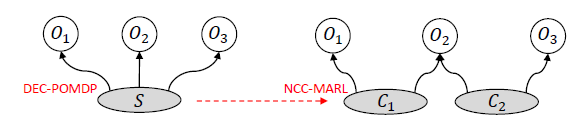• 如果邻域中的智能体可以将$C$进行完整的表征，那么它们最终会达到认知一致性，即学到的认知表征$\widehat{C_{i}}$要与真实的认知隐变量$C$相近

$p\left(C | o_{i}\right)=\frac{p\left(o_{i} | C\right) p(C)}{p\left(o_{i}\right)}=\frac{p\left(o_{i} | C\right) p(C)}{\int p(x | C) p(C) d C}$

$\min K L\left(q\left(C | o_{i}\right) \| p\left(C | o_{i}\right)\right)$

$\max \mathbb{E}_{q\left(C | o_{i}\right)} \log p\left(o_{i} | C\right)-K L\left(q\left(C | o_{i}\right) \| p(C)\right)$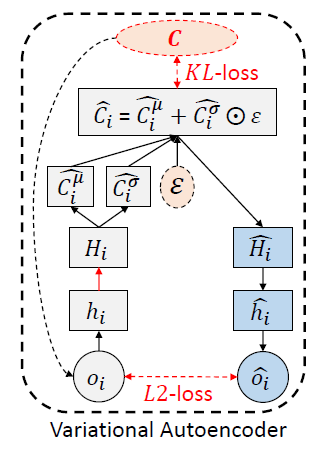$\min L 2\left(o_{i}, \widehat{o_{i}} ; w\right)+K L\left(q\left(\widehat{C_{i}} | o_{i} ; w\right) \| p(C)\right)$

#### 2.1.4 NCC-Q的训练

• TD loss：鼓励所以智能体最大化$Q_{t o t a l}$，即\begin{aligned} L^{t d}(w) &=\mathbb{E}_{\left(\vec{o}, \vec{a}, r, \vec{o}^{\prime}\right)}\left[\left(y_{t o t a l}-Q_{t o t a l}(\vec{o}, \vec{a} ; w)\right)^{2}\right] \\ y_{t o t a l} &=r+\gamma \max _{\vec{a}^{\prime}} Q_{t o t a l}\left(\vec{o}^{\prime}, \vec{a}^{\prime} ; w^{-}\right) \end{aligned}

• 认知不一致loss：$L_{i}^{c d}(w)=\mathbb{E}_{o_{i}}\left[L 2\left(o_{i}, \widehat{o_{i}} ; w\right)+K L\left(q\left(\widehat{C_{i}} | o_{i} ; w\right) \| p(C)\right)\right]$ 因此，总的loss则为：$L^{\text {total}}(w)=L^{t d}(w)+\alpha \Sigma_{i=1}^{N} L_{i}^{c d}(w)$ 但是在实际中，我们无法获得真实的认知隐变量$C$及其分布$p(C)$，而且如何为每个邻域选择一个$p(C)$也是一件很复杂的事情。因此，本文使用每个智能体及其邻域内其他智能体的认知来作为替代，即让同一邻域内智能体的认知达到一致即可，而不规定它们需要达到怎样的认知，即替换认知不一致loss为：

\begin{aligned} L_{i}^{c d}(w)=& \mathbb{E}_{o_{i}}\left[L 2\left(o_{i}, \widehat{\partial}_{i} ; w\right)+K L\left(q\left(\widehat{C}_{i} | o_{i} ; w\right) \| p(C)\right)\right] \\ \approx & \mathbb{E}_{o_{i}}\left[L 2\left(o_{i}, \widehat{o}_{i} ; w\right)+\frac{1}{|N(i)|} \Sigma_{j \in N(i)} K L\left(q\left(\widehat{C_{i}} | o_{i} ; w\right) \| q\left(\widehat{C_{j}} | o_{j} ; w\right)\right)\right] \end{aligned}

### 2.2 NCC-AC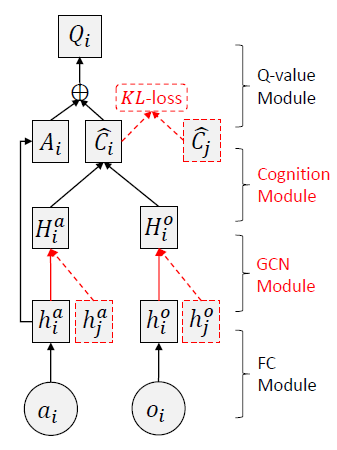\begin{aligned} L_{i}^{\text {total}}\left(w_{i}\right)=& L_{i}^{t d}\left(w_{i}\right)+\alpha L_{i}^{c d}\left(w_{i}\right) \\ L_{i}^{t d}\left(w_{i}\right)=& \mathbb{E}_{\left(o_{i}, \vec{o}_{-i}, a_{i}, \vec{a}_{-i}, r, o_{i}^{\prime}, \vec{o}_{-i}^{\prime}\right) \sim D}\left[\left(\delta_{i}\right)^{2}\right] \\ \delta_{i}=& r+\left.\gamma Q_{i}\left(\left\langle o_{i}^{\prime}, a_{i}^{\prime}\right\rangle, \vec{o}_{-i}^{\prime}, \vec{a}_{-i}^{\prime} ; w_{i}^{-}\right)\right|_{a_{j}^{\prime}=\mu_{\theta}-\left(o_{j}^{\prime}\right)} - Q_{i}\left(\left\langle o_{i}, a_{i}\right\rangle, \vec{o}_{-i}, \vec{a}_{-i} ; w_{i}\right) \\L_{i}^{c d}\left(w_{i}\right) \approx & \mathbb{E}_{o_{i}}\left[L 2\left(o_{i}, \widehat{o_{i}} ; w_{i}\right)+L 2\left(a_{i}, \widehat{a}_{i} ; w_{i}\right)+\frac{1}{|N(i)|} \sum_{j \in N(i)} K L\left(q\left(\widehat{C_{i}} | o_{i}, a_{i} ; w_{i}\right) \| q\left(\widehat{C_{j}} | o_{j}, a_{j} ; w_{j}\right)\right)\right] \end{aligned}

## 三、实验内容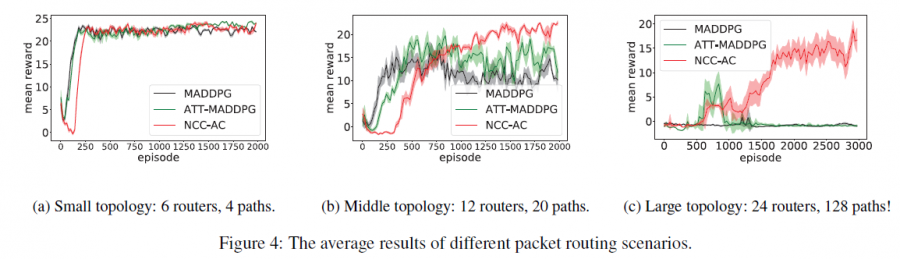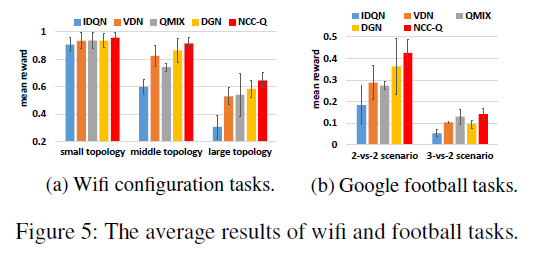## 四、缺点

• 划分邻域的方式还是通过距离
• 不同邻域之间的智能体没有显式进行弱协调。

## 五、优点

• 怎么对所有智能体进行动态、自适应划域？
• 怎么对同一邻域内的智能体进行强协调？
• 怎么对不同邻域内的智能体进行适当的弱协调？

##### Share